﻿ 带有忆阻器的Morris-Lecar神经元模型的放电模式分析和电路实现

# 带有忆阻器的Morris-Lecar神经元模型的放电模式分析和电路实现Firing Pattern Analysis and Circuit Implementation of Morris-Lecar Neuron Model with Memristor

Abstract: Improving neurons and studying their electrical activities according to the real biophysical envi-ronment are of great significance for human cognitive brain activity and neural behavior. Mor-ris-Lecar (M-L) is a representative neuron model based on ion channel, but it does not consider the effect of electromagnetic induction. The complex transmembrane motion of ions on the neuronal cell membrane can establish time-varying electromagnetic fields and affect the transition firing patterns of neurons. In this paper, a threshold memristor is used to describe the electromagnetic induction and magnetic field effects of neuron cell membrane ion exchangeto improve the neuron model, and a memristive Morris-Lecar neuron model is proposed. Numerical simulation confirms that different intensities of electromagnetic fields can produce distinct pattern transition in elec-trical activities of the neuron. From the perspective of neuron’s interspike interval (ISI), the ISIs bi-furcation in the multi-parameter planes, ISIs firing periods, the variance of ISIs and other methods are used to find the trend of the neuron firing pattern transition. Finally, a practical circuit which can reproduce the biological characteristics of neurons is designed by using Multisim, which can help to understand the mechanism of neuronal firing switching.

1. 引言

2. 带有忆阻器的M-L神经元模型的建立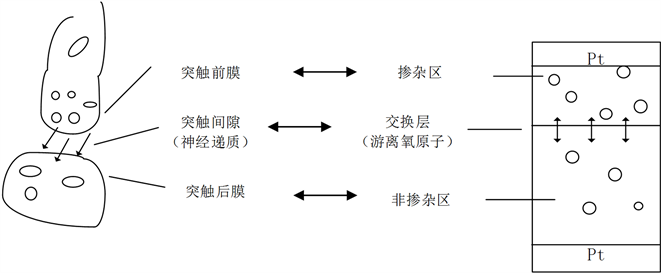Figure 1. Schematics of simplified synapse and memristor

$W\left(\phi \right)=\frac{\text{d}q\left(\phi \right)}{\text{d}\phi }=-m\mathrm{tanh}\left(\phi \right),$ (1)

$\begin{array}{l}C\frac{\text{d}V}{\text{d}t}=-u-{g}_{l}\left(V-{V}_{l}\right)-{g}_{k}\omega \left(V-{V}_{k}\right)-{g}_{Ca}{m}_{\infty }\left(V\right)\left(V-{V}_{ca}\right),\\ \frac{\text{d}\omega }{\text{d}t}=\lambda \left(V\right)\left({\omega }_{\infty }\left(V\right)-\omega \right),\\ \frac{\text{d}u}{\text{d}t}=a\left(0.2+V\right),\end{array}$ (2)

$\left\{\begin{array}{l}{m}_{\infty }\left(V\right)=0.5\left(1+\mathrm{tanh}\frac{V-{v}_{1}}{{v}_{2}}\right),\hfill \\ {\omega }_{\infty }\left(V\right)=0.5\left(1+\mathrm{tanh}\frac{V-{v}_{3}}{{v}_{4}}\right),\hfill \\ \lambda \left(V\right)=\frac{1}{3}\mathrm{cosh}\frac{V-{v}_{3}}{2{v}_{4}}.\hfill \end{array}$ (3)

$\begin{array}{l}C\frac{\text{d}V}{\text{d}t}=-u-m\mathrm{tanh}\left(\phi \right)V-{g}_{l}\left(V-{V}_{l}\right)-{g}_{k}\omega \left(V-{V}_{k}\right)-{g}_{Ca}{m}_{\infty }\left(V\right)\left(V-{V}_{ca}\right),\\ \frac{\text{d}\omega }{\text{d}t}=\lambda \left(V\right)\left({\omega }_{\infty }\left(V\right)-\omega \right),\\ \frac{\text{d}u}{\text{d}t}=a\left(0.2+V\right),\\ \frac{\text{d}\phi }{\text{d}t}=-V,\end{array}$ (4)

$\left\{\begin{array}{l}{m}_{\infty }\left(V\right)=0.5\left(1+\mathrm{tanh}\frac{V-{v}_{1}}{{v}_{2}}\right),\hfill \\ {\omega }_{\infty }\left(V\right)=0.5\left(1+\mathrm{tanh}\frac{V-{v}_{3}}{{v}_{4}}\right),\hfill \\ \lambda \left(V\right)=\frac{1}{3}\mathrm{cosh}\frac{V-{v}_{3}}{2{v}_{4}}.\hfill \end{array}$ (5)

$i\text{'}=\frac{\text{d}q\left(\phi \right)}{\text{d}t}=\frac{\text{d}q\left(\phi \right)}{\text{d}\phi }\frac{\text{d}\phi }{\text{d}t}=W\left(\phi \right)v=-m\mathrm{tanh}\left(\phi \right)v,$ (6)

3. 带有忆阻器的神经元模型放电模式趋势分析

3.1. 膜电容和磁感应强度双参数下神经元放电模式变化趋势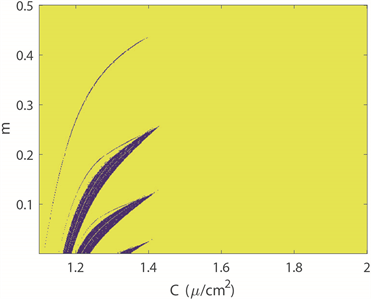(a) 膜电位周期性分布图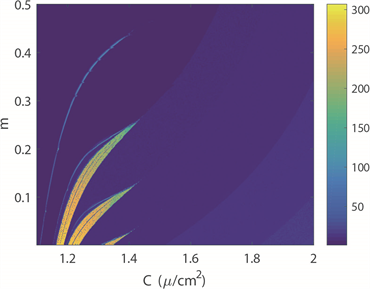(b) 膜电位放电周期数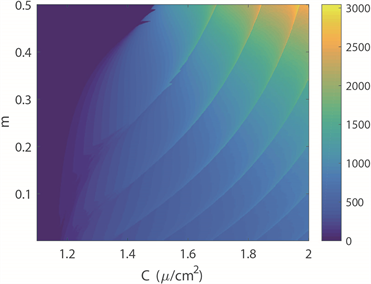(c) ISIs方差分布图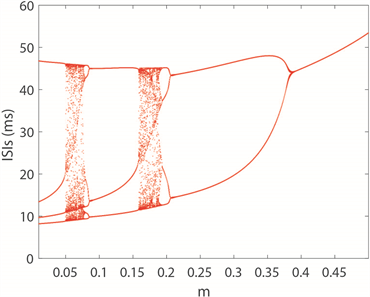(d) 关于参数m ISIs分岔图

Figure 2. Transition of neuron firing patterns under C and m parameters

1) 当 $C\in \left[1.1,\text{\hspace{0.17em}}1.168\right]$ 时，带有忆阻器的四维M-L神经元系统随着磁感应强度增加全部呈现简单的尖峰放电模式，并且放电节律几乎没有变化。

2) 当 $C\in \left[1.168,\text{\hspace{0.17em}}1.434\right]$ 时，该系统随着磁感应强度增加呈现出复杂的放电模式转变，即由周期性簇放电到混沌放电模式再到尖峰放电，神经元放电节律逐渐变慢。

3) 当 $C\in \left[1.434,\text{\hspace{0.17em}}2\right]$ 时，该系统随着磁感应强度增加全部呈现簇放电模式并且簇中尖峰数量呈逐渐减少趋势，神经元放电节律逐渐减慢。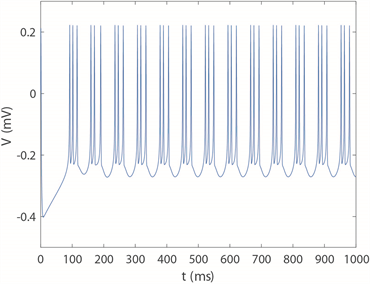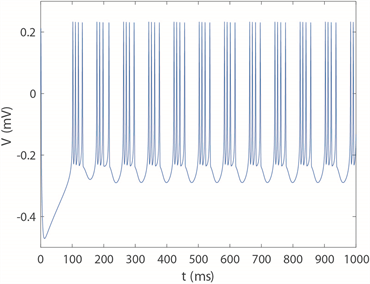(a) 周期4簇放电模式( $m=0.028$ )(b) 周期3簇放电模式( $m=0.125$ )

(c) 周期2簇放电模式( $m=0.227$ )(d) 周期2尖峰放电模式( $m=0.365$ )

Figure 3. Fixed membrane capacitance $C=1.3\text{\hspace{0.17em}}\mu F/c{m}^{2}$ , the M-L neuron membrane potential timing diagram

3.2. 磁感应强度和时间尺度因子双参数下神经元放电模式变化趋势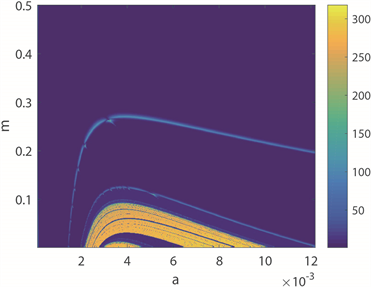(a) 膜电位周期性分布图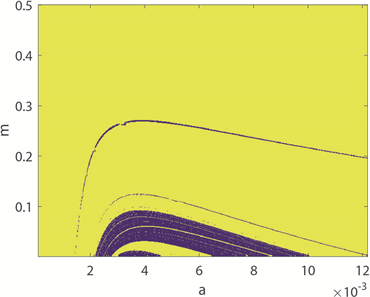(b) 膜电位放电周期数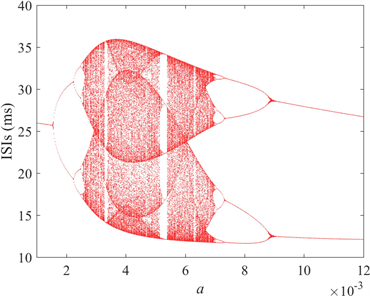(c) ISIs方差分布图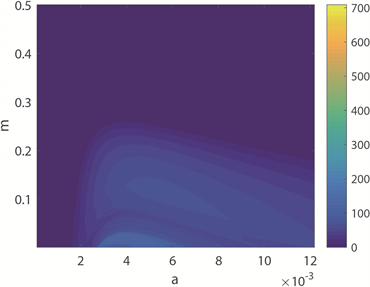(d) 关于参数a的ISIs分岔图

Figure 4. Transition of neuron firing patterns under a and m parameters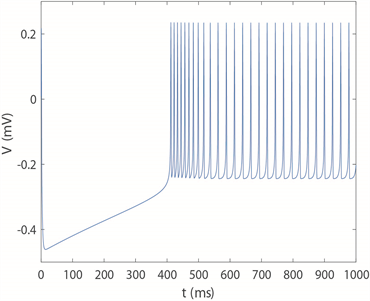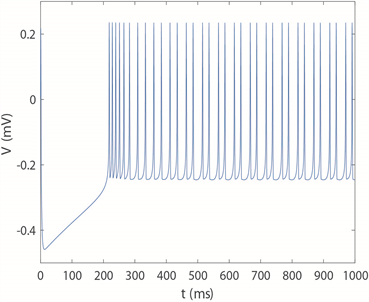(a) 周期1尖峰放电模式( $a=0.001$ )(b) 周期2尖峰放电模式( $a=0.002$ )

(c) 混沌放电模式( $a=0.004$ )(d) 周期3簇放电模式( $a=0.008$ )

(e) 周期2簇放电模式( $a=0.01$ )

Figure 5. The neuron membrane potential timing diagram with fixed magnetic induction intensity $m=0.05$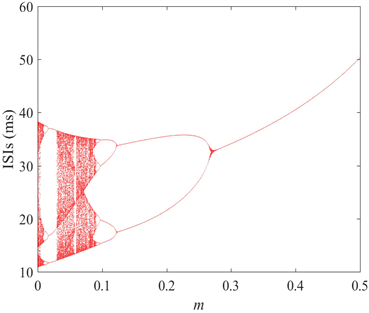(a) 周期1尖峰放电模式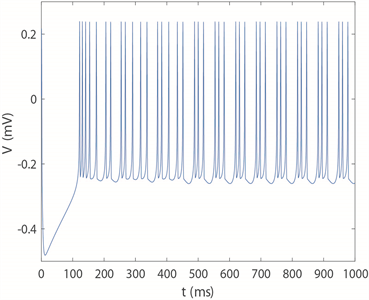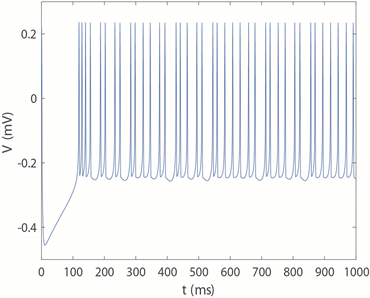(b) 周期3簇放电模式( $m=0.02$ ) (c) 混沌放电模式( $m=0.05$ )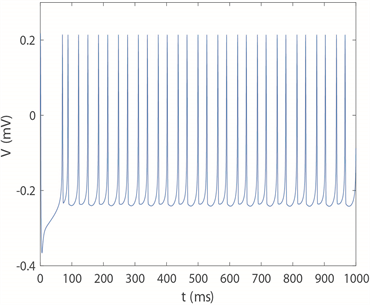(d) 周期2簇放电模式( $m=0.15$ ) (e) 周期2尖峰放电模式( $m=0.25$ )

Figure 6. Fixing the time scale factor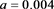, the neuron membrane potential timing diagram

4. 带有忆阻器的M-L神经元的系统实现

4.1. 双曲正切函数关系电路实现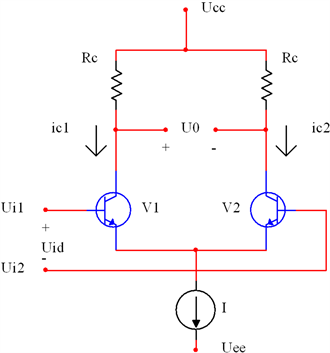Figure 7. Constant current source differential amplifier circuit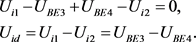(7)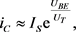(8)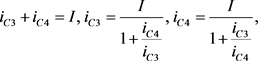(9)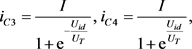(10)(11)(12)

4.2. 双曲余弦函数关系电路的实现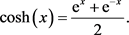(13)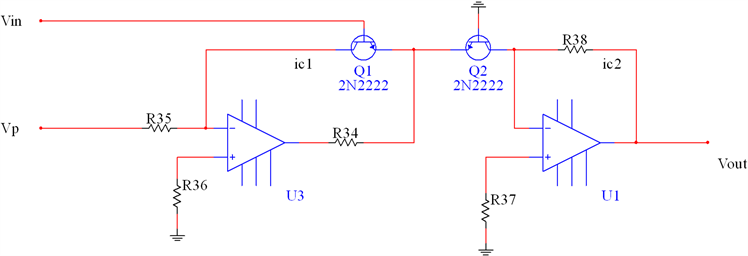Figure 8. Exponential function circuit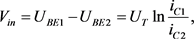(14)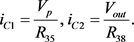(15)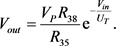(16)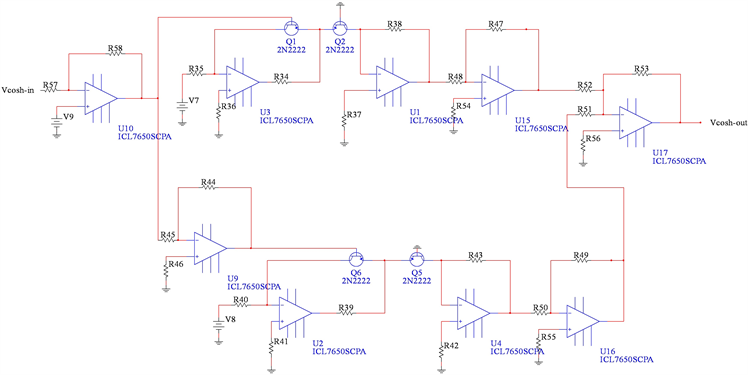Figure 9. Circuit unit of the hyperbolic cosine function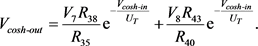(17)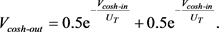(18)

4.3. 带有忆阻器的M-L神经元模型的电路实现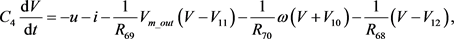(19)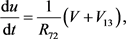(20)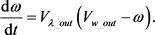(21)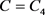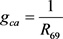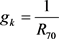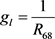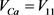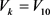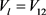，i对应于方框A忆阻器输出电流。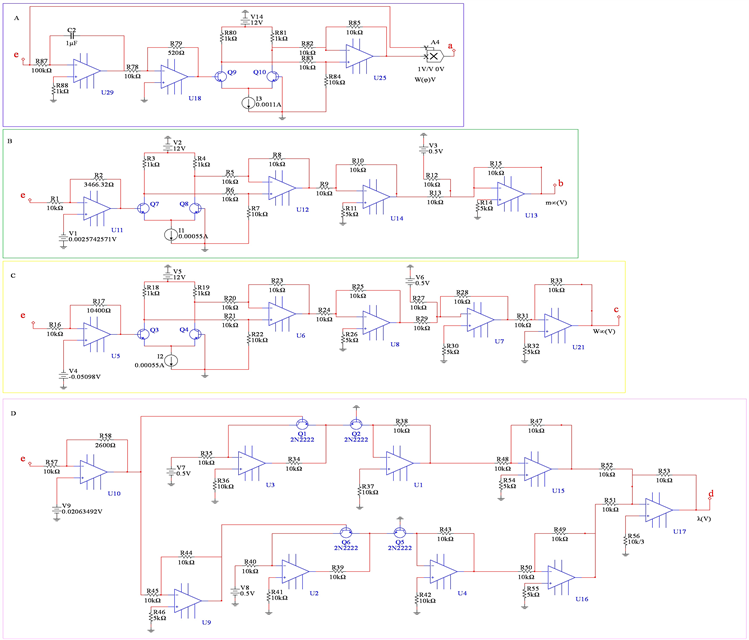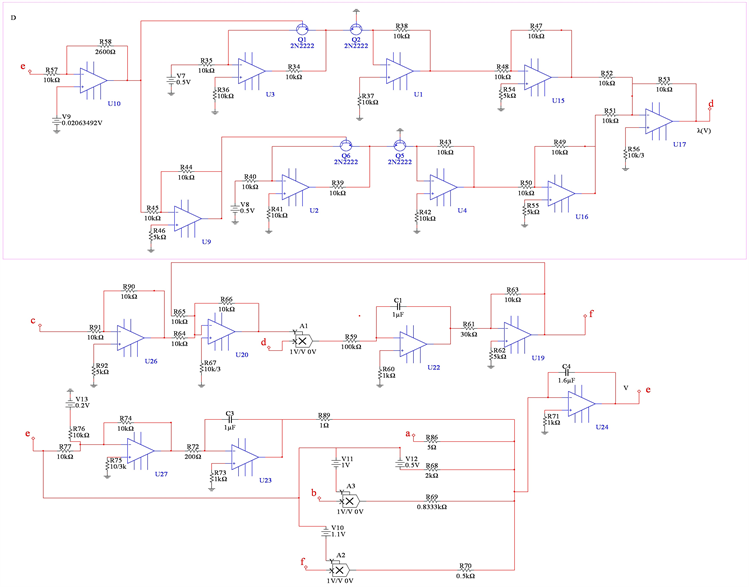Figure 10. Complete circuit realization of 4D neuron system with memristor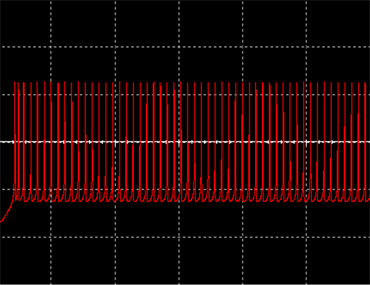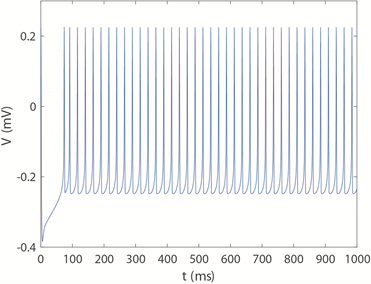(a) 当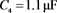时电路实验结果 (b) 当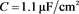时数值仿真结果

(c) 当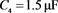时电路实验结果 (d) 当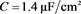时数值仿真结果

Figure 11. Comparison diagram between experimental results and numerical simulation

5. 总结

 Bertram, R., Butte, M.J., Kiemel, T. and Sherman, A. (1995) Topological and Phenomenological Classification of Burst-ing Oscillations. Bulletin of Mathematical Biology, 57, 413-439.
https://doi.org/10.1016/S0092-8240(05)81776-8

 Chay, T.R., Fan, Y.S. and Lee, Y.S. (1995) Bursting Spiking Chaos Fractals and Universality in Biological Rhythms. International Journal of Bifurcation and Chaos, 5, 595-635.
https://doi.org/10.1142/S0218127495000491

 Rabinovich, M.I., Abarbanel, H.D.I., Huerta, R. and Selverston, A.I. (1997) Self-Regularization of Chaos in Neural Systems: Experimental and Theoretical Results. IEEE Transactions on Circuits and Systems I, 44, 997-1005.
https://doi.org/10.1109/81.633889

 Ermentrout, G.B. and Terman, D. (2010) Foundations of Mathematical Neuroscience. Interdisciplinary Applied Mathematics, Springer, Berlin.

 Hodgkin, A.L. and Huxley, A.F. (1952) A Quantitative Description of Membrane Current and Its Application to Conduction and Excitation in Nerve. The Journal of Physiology, 117, 500-544.
https://doi.org/10.1113/jphysiol.1952.sp004764

 Morris, C. and Lecar, H. (1981) Voltage Oscillations in the Barnacle Giant Muscle Fiber. Biophysical Journal, 35, 193-213.
https://doi.org/10.1016/S0006-3495(81)84782-0

 Rinzel, J. and Ermentrout, G.B. (1989) Analysis of Neural Excitability and Oscillations. Methods in Neuronal Modeling. MIT Press, Cambridge.

 Tsumoto, K., Kitajima, H., Yoshinaga, T., Aihara, K. and Kawakami, H. (2006) Bifurcations in Morris-Lecar Neuron Model. Neurocomputing, 69, 293-316.
https://doi.org/10.1016/j.neucom.2005.03.006

 Shi, M. and Wang, Z. (2014) Abundant Bursting Pat-terns of a Fractional-Order Morris-Lecar Neuron Model. Communications in Nonlinear Science and Numerical Simula-tion, 19, 1956-1969.
https://doi.org/10.1016/j.cnsns.2013.10.032

 Izhikevich, E.M. (2000) Neural Excitability, Spiking and Bursting. International Journal of Bifurcation and Chaos, 10, 1171-1266.
https://doi.org/10.1142/S0218127400000840

 Wang, H., Lu, Q. and Wang, Q. (2005) Generation of Firing Rhythm Patterns and Synchronization in the Morris-Lecar Neuron Model. International Journal of Nonlinear Sciences and Numerical Simulation, 6, 7-12.
https://doi.org/10.1515/IJNSNS.2005.6.1.7

 Wang, H., Lu, Q. and Zheng, Y. (2009) Complex Dynamics of Neuron Models: Bifurcation and Coding. Journal of Dynamics and Control, 3, 7-10.

 Upadhyay, R.K. and Mondal, A. (2017) Synchronization of Bursting Neurons with a Slowly Varying d. c. Current. Chaos, Solitons and Fractals, 99, 195-208.
https://doi.org/10.1016/j.chaos.2017.03.063

 Song, X., Wang, H. and Chen, Y. (2019) Au-tapse-Induced Firing Patterns Transitions in the Morris-Lecar Neuron Model. Nonlinear Dynamics, 96, 2341-2350.
https://doi.org/10.1007/s11071-019-04925-7

 Chua, L. (1997) Memristor—The Missing Circuit Element. IEEE Transactions on Circuit Theory, 18, 507-519.
https://doi.org/10.1109/TCT.1971.1083337

 Strukov, D.B., Snider, G.S., Stewart, D.R. and Williams, R.S. (2008) The Missing Memristor Found. Nature, 453, 80-83.
https://doi.org/10.1038/nature06932

 Lv, M., Wang, C., Ren, G., Ma, J. and Song, X. (2016) Model of Electrical Activity in a Neuron under Magnetic Flow Effect. Nonlinear Dynamics, 85, 1479-1490.
https://doi.org/10.1007/s11071-016-2773-6

 Lv, M. and Ma, J. (2016) Multiple Modes of Electrical Activities in a New Neuron Model under Electromagnetic Radiation. Neurocomputing, 205, 375-381.
https://doi.org/10.1016/j.neucom.2016.05.004

 Bao, H., Hu, A., Liu, W. and Bao, B. (2019) Hidden Bursting Firings and Bifurcation Mechanisms in Memristive Neuron Model with Threshold Electromagnetic Induction. IEEE Transactions on Neural Networks and Learning Systems, 31, 502-511.
https://doi.org/10.1109/TNNLS.2019.2905137

 Han, I.S. (2006) Mixed-Signal Neuron-Synapse Implementa-tion for Large-Scale Neural Network. Neurocomputing, 69, 1860-1867.
https://doi.org/10.1016/j.neucom.2005.11.013

 Sun, X., Perc, M. and Kurths, J. (2018) Fast Regular Firings Induced by Intra- and Inter-Time Delays in Two Clustered Neuronal Networks. Chaos, 28, Article ID: 106310.
https://doi.org/10.1063/1.5037142

 Ahmadi, A. and Gomar, S. (2014) Digital Multiplierless Implementation of Biological Adaptive-Exponential Neuron Model. IEEE Transactions on Circuits and Systems I, 61, 1206-1219.
https://doi.org/10.1109/TCSI.2013.2286030

 Silver, R., Boahen, K., Grillner, S., Kopell, N. and Olsen, K.L. (2007) Neurotech for Neuroscience: Unifying Concepts, Organizing Principles and Emerging Tools. Journal of Neuro-science, 27, 11807-11819.
https://doi.org/10.1523/JNEUROSCI.3575-07.2007

 Wu, X., Ma, J., Yuan, L. and Liu, Y. (2014) Simulating Electric Activities of Neurons by Using PSPICE. Nonlinear Dynamics, 75, 113-126.
https://doi.org/10.1007/s11071-013-1053-y

 Behdad, R., Binczak, S., Dmitrichev, A.S., Nekorkin, V.I. and Bilbault, J.M. (2014) Artificial Electrical Morris-Lecar Neuron. IEEE Transactions on Neural Networks and Learning Systems, 26, 1875-1884.
https://doi.org/10.1109/TNNLS.2014.2360072

 Hu, X., Liu, C., Liu, L., Ni, J. and Li, S. (2016) An Electronic Implementation for Morris-Lecar Neuron Model. Nonlinear Dynamics, 4, 2317-2332.
https://doi.org/10.1007/s11071-016-2647-y

Top